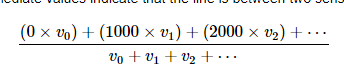`````` unsigned int position = robot.readLine(sensors, IR_EMITTERS_ON);

``````

I mean code for used measure sensor output in single umerical result

Hello.

It is not entirely clear to me what specific product you are referring to (i.e. where that code came from) or what exactly you are asking, but it sounds like you want to know how the `readLine()` function takes all of the line sensor readings and outputs a single value.

You can find details about that in the documentation for the `readLineBlack()` function in our Arduino library for our QTR reflectance sensors. (Please note that our updated QTR library separates the `readLine()` function into two: one for a black line and one for a white line). Essentially, it just takes a weighted average of the sensor indices. If you want to see the code for it, you can look through the `readLinePrivate()` function defined on line 644 in QTRSensors.cpp.

Brandon

1 Like

Hello BrandonM

Sorry for less explain

We have old 3pi robot and old 8QTRC sensor

we are teaching robotics for children using them and Arduino code

Our students are asking about how to take single value using code

https://pololu.github.io/qtr-sensors-arduino/_q_t_r_sensors_8cpp_source.html

I tired to understand the codes in the above library how to extract specific code for read the single value but I can not understand it as lot of codes are there which is can not understand

Can you please give us specific code sample for the teaching purpose

`````` switch (_type)
{
case QTRType::RC:
for (uint8_t i = start; i < _sensorCount; i += step)
{
sensorValues[i] = _maxValue;
// make sensor line an output (drives low briefly, but doesn't matter)
pinMode(_sensorPins[i], OUTPUT);
// drive sensor line high
digitalWrite(_sensorPins[i], HIGH);
}

delayMicroseconds(10); // charge lines for 10 us

{
// disable interrupts so we can switch all the pins as close to the same
// time as possible
noInterrupts();

// record start time before the first sensor is switched to input
// (similarly, time is checked before the first sensor is read in the
// loop below)
uint32_t startTime = micros();
uint16_t time = 0;

for (uint8_t i = start; i < _sensorCount; i += step)
{
// make sensor line an input (should also ensure pull-up is disabled)
pinMode(_sensorPins[i], INPUT);
}

interrupts(); // re-enable

while (time < _maxValue)
{
// disable interrupts so we can read all the pins as close to the same
// time as possible
noInterrupts();

time = micros() - startTime;
for (uint8_t i = start; i < _sensorCount; i += step)
{
if ((digitalRead(_sensorPins[i]) == LOW) && (time < sensorValues[i]))
{
// record the first time the line reads low
sensorValues[i] = time;
}
}

interrupts(); // re-enable
}
}
return;
``````

No idea about duty of _maxValue in the above code

Can you please explain operation of below codes it is grate thanks

`````` time = micros() - startTime;
for (uint8_t i = start; i < _sensorCount; i += step)
{
if ((digitalRead(_sensorPins[i]) == LOW) && (time < sensorValues[i]))
{
// record the first time the line reads low
sensorValues[i] = time;
}
}
``````

Thanks again

Hello.

Unfortunately, I am still not entirely sure what you are asking. It sounds like you might be asking how to look at the individual sensor readings after calling one of the `readLine()` functions. If that’s the case, you can access them from the array passed to the `readLine()` function as the first argument. For example, if you use the line of code you posted about in your original question (i.e. `unsigned int position = robot.readLine(sensors, IR_EMITTERS_ON);`) the individual sensor readings will be stored in the `sensors` array (i.e. `sensors` will hold the reading for sensor 0 and so on).

``````time = micros() - startTime;
for (uint8_t i = start; i < _sensorCount; i += step)
{
if ((digitalRead(_sensorPins[i]) == LOW) && (time < sensorValues[i]))
{
// record the first time the line reads low
sensorValues[i] = time;
}
}
``````

Before this code is called, capacitors on the output node are charged by driving the line high and that snipped of code is timing how long it takes the output voltage for each sensor to decay. The brighter the reflectance, the faster the voltage will decay.

Brandon

Can you please explain the operation of following code

`````` if ((digitalRead(_sensorPins[i]) == LOW) && (time < sensorValues[i]))
``````

and also please provide the code sniper for the following calcutationThe capacitor is charged and the code is then timing how long it takes to discharge. When it is discharged it will read as `LOW` so this code stores away the time that it first sees the sensor read as low. The `time < sensorValues[i])` part of the conditional makes sure that `sensorValues[i]` is only updated the one time since once it reads `LOW`, it will continue to read as `LOW`.